Integration by parts

Chapter 7 Class 12 Integrals
Concept wise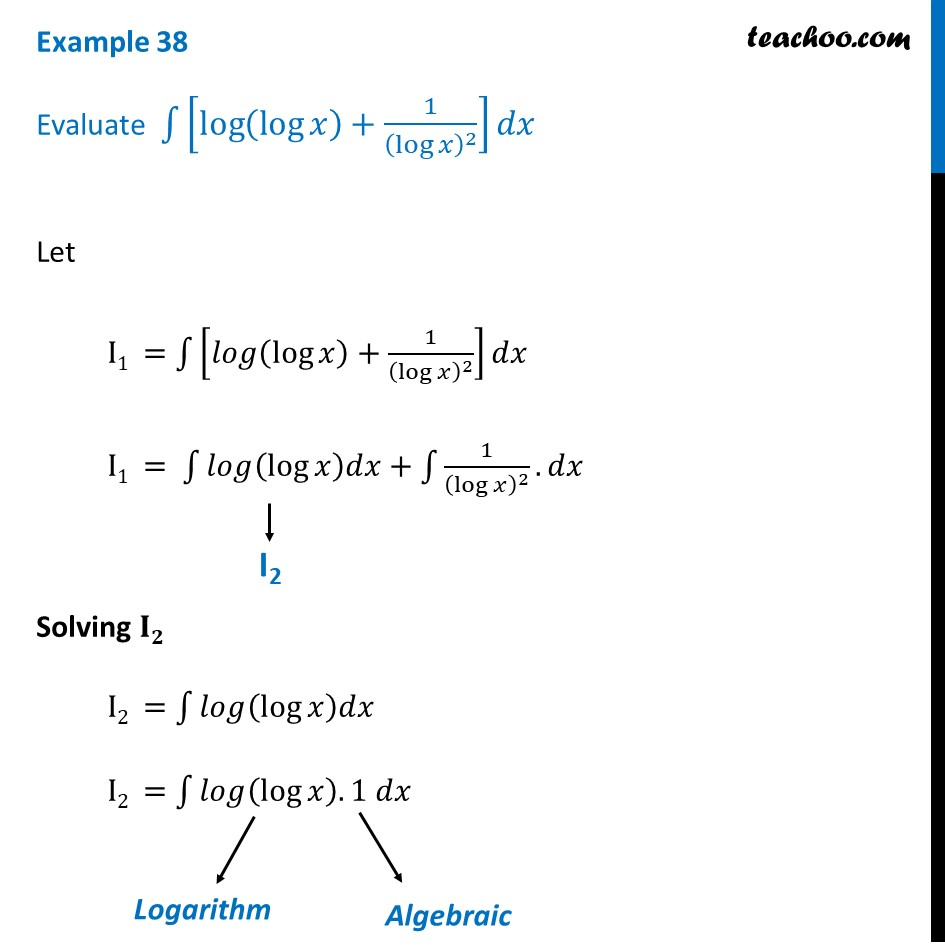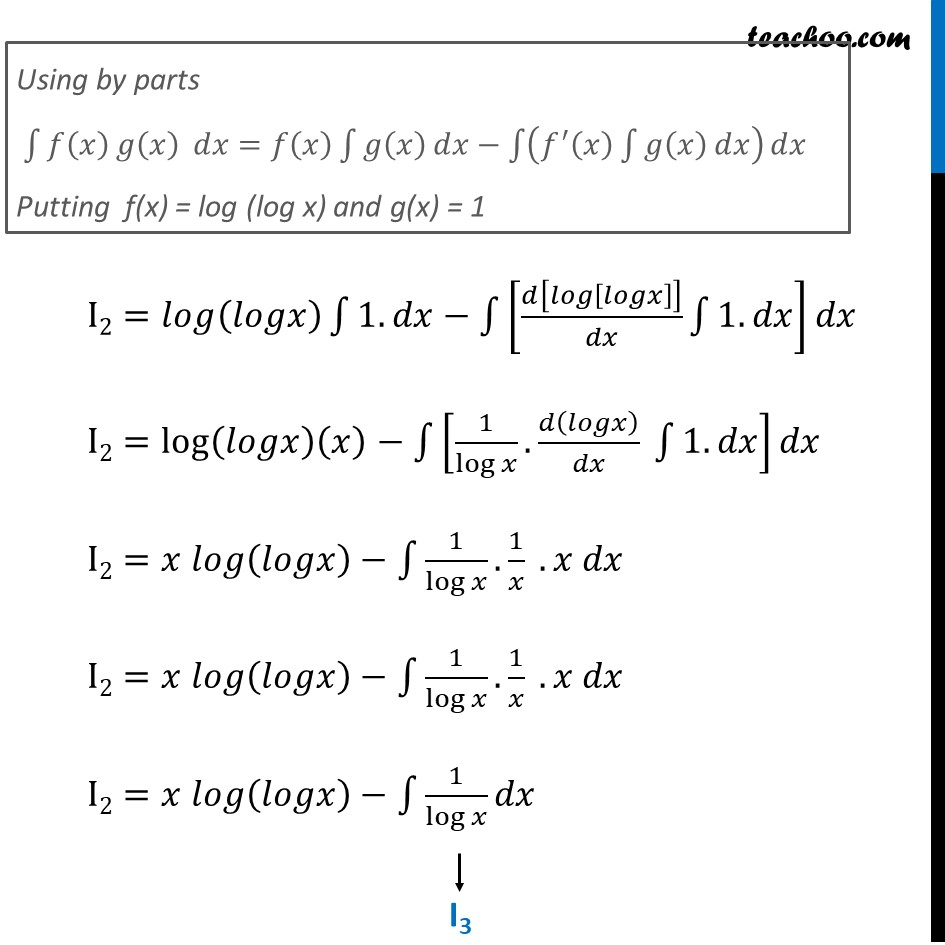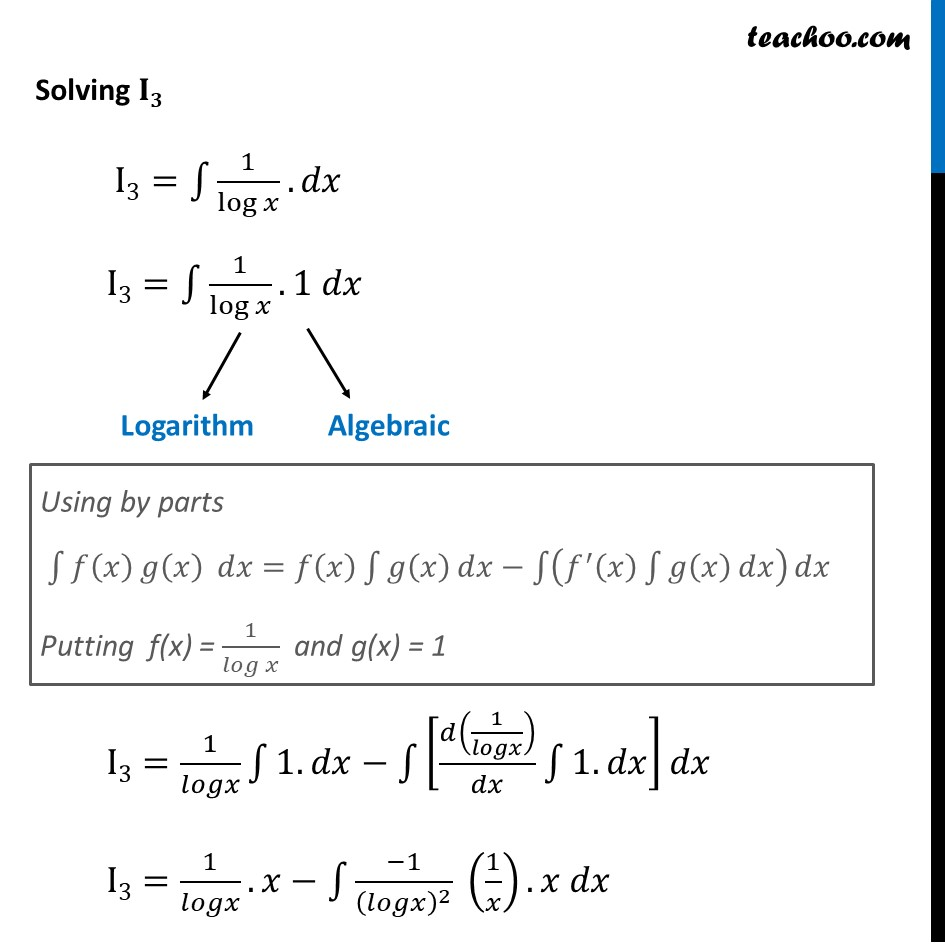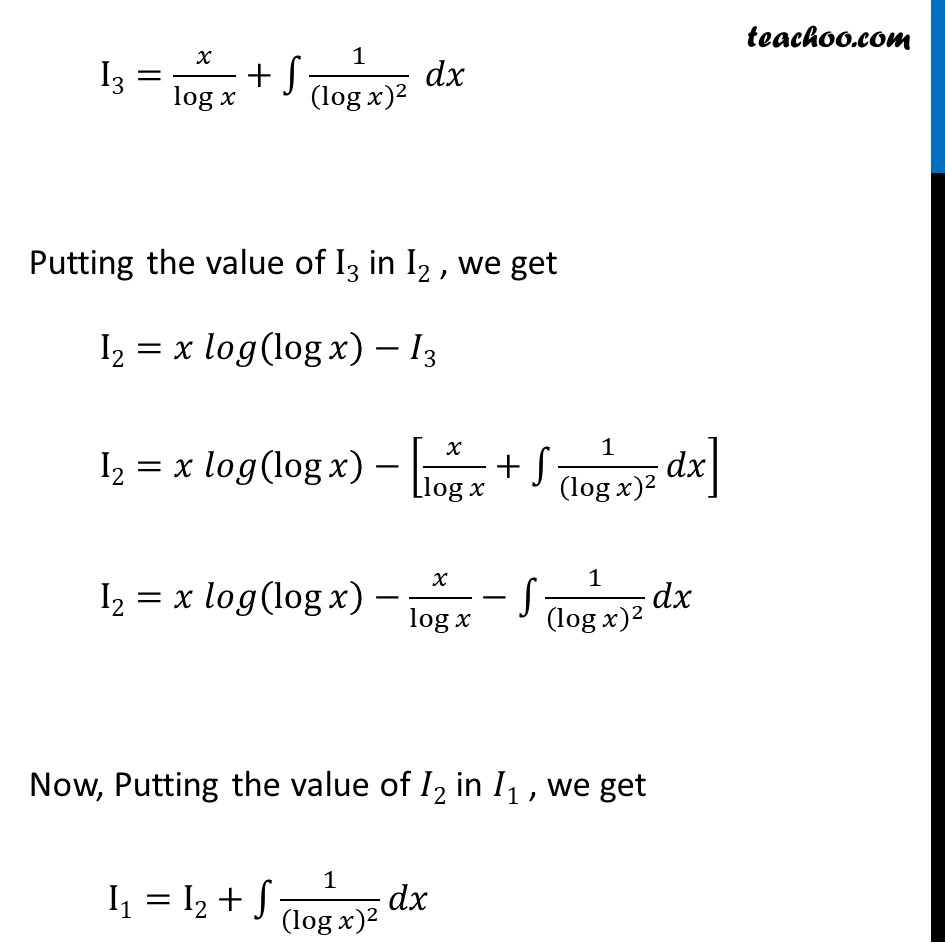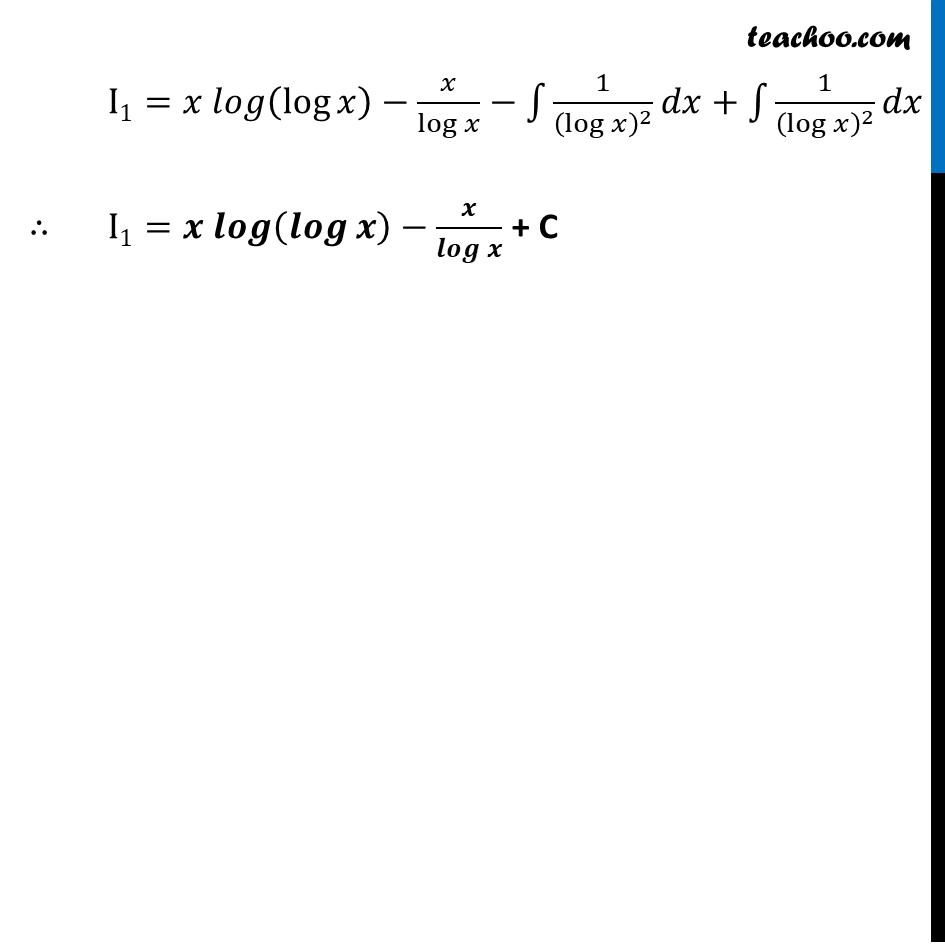Learn in your speed, with individual attention - Teachoo Maths 1-on-1 Class

### Transcript

Example 38 Evaluate ∫1▒[log⁡(log⁡𝑥 )+1/(log⁡𝑥 )^2 ] 𝑑𝑥 Let I1 =∫1▒[𝑙𝑜𝑔(log⁡𝑥 )+1/(log⁡𝑥 )^2 ]𝑑𝑥 I1 = ∫1▒〖𝑙𝑜𝑔(log⁡𝑥 )𝑑𝑥+∫1▒〖1/(log⁡𝑥 )^2 .𝑑𝑥〗〗 Solving 𝐈𝟐 I2 =∫1▒𝑙𝑜𝑔(log⁡𝑥 )𝑑𝑥 I2 =∫1▒〖𝑙𝑜𝑔(log⁡𝑥 ).1 𝑑𝑥〗 Let I1 =∫1▒[𝑙𝑜𝑔(log⁡𝑥 )+1/(log⁡𝑥 )^2 ]𝑑𝑥 I1 = ∫1▒〖𝑙𝑜𝑔(log⁡𝑥 )𝑑𝑥+∫1▒〖1/(log⁡𝑥 )^2 .𝑑𝑥〗〗 Solving 𝐈𝟐 I2 =∫1▒𝑙𝑜𝑔(log⁡𝑥 )𝑑𝑥 I2 =∫1▒〖𝑙𝑜𝑔(log⁡𝑥 ).1 𝑑𝑥〗 I2=𝑙𝑜𝑔(𝑙𝑜𝑔𝑥) ∫1▒〖1.𝑑𝑥−∫1▒[𝑑[𝑙𝑜𝑔[𝑙𝑜𝑔𝑥]]/𝑑𝑥 ∫1▒〖1.𝑑𝑥〗] 〗 𝑑𝑥 I2=log(𝑙𝑜𝑔𝑥)(𝑥)−∫1▒[1/log⁡𝑥 .𝑑(𝑙𝑜𝑔𝑥)/𝑑𝑥 ∫1▒〖1.𝑑𝑥〗]𝑑𝑥 I2=𝑥 𝑙𝑜𝑔(𝑙𝑜𝑔𝑥)−∫1▒〖1/log⁡𝑥 .1/𝑥 .𝑥 𝑑𝑥〗 I2=𝑥 𝑙𝑜𝑔(𝑙𝑜𝑔𝑥)−∫1▒〖1/log⁡𝑥 .1/𝑥 .𝑥 𝑑𝑥〗 I2=𝑥 𝑙𝑜𝑔(𝑙𝑜𝑔𝑥)−∫1▒〖1/log⁡𝑥 𝑑𝑥〗 Solving 𝐈𝟑 I3=∫1▒〖1/log⁡𝑥 .𝑑𝑥〗 I3=∫1▒〖1/log⁡𝑥 .1 𝑑𝑥〗 I3=1/𝑙𝑜𝑔𝑥 ∫1▒〖1.𝑑𝑥−∫1▒[𝑑(1/𝑙𝑜𝑔𝑥)/𝑑𝑥 ∫1▒〖1.𝑑𝑥〗] 〗 𝑑𝑥 I3=1/𝑙𝑜𝑔𝑥.𝑥−∫1▒〖(−1)/(𝑙𝑜𝑔𝑥)^2 (1/𝑥).𝑥 𝑑𝑥〗 I3=𝑥/log⁡𝑥 +∫1▒〖1/(log⁡𝑥 )^2 𝑑𝑥〗 Putting the value of I3 in I2 , we get I2=𝑥 𝑙𝑜𝑔(log⁡𝑥 )−𝐼3 I2=𝑥 𝑙𝑜𝑔(log⁡𝑥 )−[𝑥/log⁡𝑥 +∫1▒1/(log⁡𝑥 )^2 𝑑𝑥] I2=𝑥 𝑙𝑜𝑔(log⁡𝑥 )−𝑥/log⁡𝑥 −∫1▒〖1/(log⁡𝑥 )^2 𝑑𝑥〗 Now, Putting the value of 𝐼2 in 𝐼1 , we get I1=I2+∫1▒〖1/(log⁡𝑥 )^2 𝑑𝑥〗 I1=𝑥 𝑙𝑜𝑔(log⁡𝑥 )−𝑥/log⁡𝑥 −∫1▒〖1/(log⁡𝑥 )^2 𝑑𝑥〗+∫1▒〖1/(log⁡𝑥 )^2 𝑑𝑥〗 ∴ I1=𝒙 𝒍𝒐𝒈(𝒍𝒐𝒈⁡𝒙 )−𝒙/𝒍𝒐𝒈⁡𝒙 + C